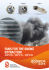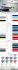## Transcription

```MAC 2233 Final Exam Review
Instructions: The final exam will consist of 10 questions and be worth 150 points.
The point value for each part of each question is listed in the question, and the
total point value of each question is listed below. Only a basic calculator or
scientific calculator may be used, although a calculator is not necessary for the
exam. NO GRAPIDNG CALCULATORS OR CALCU LATORS ON A
D EV ICE (SUCH AS iPOD, CELL P HONE, ETC.) WmCH CAN B E
U SED FOR ANY P URPOSE OTHER THAN A S A CALCULATOR
WILL B E ALLOWED! The concepts and types of questions on the final exam
will be similar to the previous tests, previous reviews, and this review, although
the numbers and functions may be different on each question on the exam. The
questions on the exam will be taken from the previous four exams. Each of the
10 questions can be found in the following places:
1. Exam #1, Problem #2 or Section 1.5 (18 points)
2. Exam #2, Problem # 1 or Section 2.5 (8 points)
3. Exam #2, Problem #2 or Section 2.6 (12 points)
4. Exam #2, Problem # 5 or Section 3.1 & 3.2 (20 points)
5. Exam #2, Problem #6 or Section 3.3 (8 points)
6. Exam #3, Problem # 2 or Section 3.5 (12 points)
7. Exam #3, Problem #4 or Section 4.3 & 4.5 (20 points)
8. Exam #4, Problem #1 or Section 5.1 (16 points)
9. Exam #4, Problem #2 or Section 5.2 (16 points)
10. Exam #4, Problem #4 or Section 5.4 (20 points)
1. Find each limit. If a limit does not exist, explain why it does
not exist. (6 points each)
(a)
. vx+3-2
1I m - - - ­
x-I
x-+ l
- ~-~ ­
-
{\{ -L. _2-~ __ 0
I-l»- /-l-O
~
1r<k.felM;WA-k
-
--
--_\.(\f +J.
\
- -- .
a+d-
(b)
x 2 - 6
lim - ­
x-+-4 x-I
(c)
.
11m
x-+-2
{D
-~
x
(x
+ 2)2
~
---­
0
- ~
J.
2. Find slope of t he tangent line to the graph of f(x)
at the point (5,2) . (8 points)
-t()():: (x.~ - foX t i )'/l.
-f ' (lc.) ~ i (X>- - lox +i Y-}. (2l< -(")
r.
of' (s-j :=. ~ (SL-(.(,} rl ~ (2 (,) -"J
- -i (;}.':)-3D +1y-i
- ~(~r~ . (~) I
.. (~\
~0\ . (10 -fo) =
vx 2
-
6x + 9
3. (a) Find ~ for the function y
=
(2X2 - 3x + 4)5. (6 points)
(b) Find f(5)(x) for t he function f(x) = 2x 5 + 4x 3
points)
.f' (~\=. lOx. '1 +- 12k 2 - 2
f
\I
(>c\ ="{OK~ FZ-'1)c
·r\( ()()-: : 110 >? -\- '2- ~
-t C'" (X) ~ Zl{O)(
-
2x. (6
= 2~:;8.
4. Let f(x)
(a) Find the critical numbers. (5 points)
f'('IC)-:: 2'(~(~Xl) - (2x"-&)('{,..) =-
\2x'1
-(~x'f-'2X)
~x1.y·
4x'{ -r)2~_
_
C(~'i
~k'" 'ir(
_
;:; K,3
+~)
'Ix.'13 =
-
J\'+~ X.~ ­
-F \(l()~vwkH~ 1...'J -= 0 ·r(x)~()
X:~~::o
=i-:!.
~-::~
X"J '=- ~
~3
~~~
Lx ::. -2 )
(b) Find t he open intervals on which t he function is increasing
or decreasing. (5 points)
rr
:t
\
<cVO~
-L
r
ritl
/I.=-?> ~
:IT: : ? iQ
]I ',
~
m:
\ yO?> () r (-3) -= :rg ~ fOs
X::-\:
.f' h\"
V; ~ ~
V,Q X~\~ i\ (\'r~ -F~F
\V\CJ!'9<,i'j : (-pO, -i) V (0, o.i)
cltcr~~'4
:
(-~,O)
(c) Find the local maximum and local minimum values of the
function. (5 points)
-t'(~\ ~. ~
-11>
tiw-~"\'j
~ (-1..)
t[a1IJi
1<.
~
=
LIt-
fb(,"\M -II)
[email protected]'vt ..t
~~ - 2,
t' ~
;;l(-2')"!. -~
'i>D
a.
.1\.= - 2,
S~ .t(l<) cJ.oae~ ~ 'l"U~~lj
lnJ """'"
::- c";l(-~) - ~ _ -((, - ~ ~ - '2.~
;tl-l~2-
J(~)
-
~
tU-
it. =-
2.
=- - :5 <l yrqv)I ::: (- 2, - ))]
\."
0..
w-\\cJ a..tf'to:;'
'i>O \\-
C/NV\no+
~" (ocJ MIll< or
\louJ
- """" J
ruN
(d) Find the absolute maximum and extreme minimum of the
function on the interval [-4, -1] . (5 points)
J.(- I) - g..
+-C-1.) ~ - ~ <
Cl1i~
f()\Y\\­
~,crs+
~"'Sb\U~ ~:::
a~So)u~ ~\V\
(- ~ --S) -:: ( - \) -
s)
_
"","
5. Find the inflection points and the open intervals on which the
function f(x) = X4 - 6x 3 + 12x2 + 7x - 6 is concave upward or
concave downward. (8 points)
-f'\\Gt)~ 11x't -?:kx -t2~
::
(2(~ - 3Xf;;l.)
-::: 12 (
X-2\ (~-\)
l\-J
12- ::;'0
~
n~ 5 0\"'" Il"­
Xl:OO
+-- ±L
=-v
+:l.
x. ~ \
K::...;l \ 11'1 t(tUi()o'\
fO' ",~ :
m: < -p>s
\ fOs
r' (())=
1C~ ViUt ~::o t: -fl'
\
I,~ T
I : Y\v\t- x~o '.
]I ~
X=
~Itk K::. ?,.~
C.6v\C!A.\rt
t
\l
(r~(~)~)~
(tl= (~o»
(-,)(t ll) 4
(
6) ~ (f05)( e()~ fbS) =-pos
'f ~ (- 00) l) V (at 00) l ~tcA~ ~n ~ lLl) 6. A car rental company rent s 20 cars per week at \$100 each .
However, for each \$15 rebate offered, the rentals increase by 5
per week. It costs t he company \$10 each week to maintain each
car it rents. How much of a rebate should the company offer in
order to maximize their profit from renting cars? (12 points)
1:: 100- 3 (x-U»
1::
f
DR
IOo-")x +(00
(oO+~.i
f
X---"3
~
3
-= llao - 3x
x= ~S
c.,
V :: ltoox - 3x
2
~
-
(Dx
\' ::: lSbx _)'1."2­
-p ~ I~o- 3(~S) f -=-l (00 - -=fS­
f =~5" 'f~ I'M -h> au
.<.-
proh+
f' =-
lSD-CoX
ISD- Cof
t!£.t
=0 +bX_ I~~¥
_ O(\"~ y(){.t =_ ~OO
.. DetlO\J Py\<!. = \$~-
T£tJo~-K
-
\$ fS
J
~
.....
7. Differentiate each function. (5 points each)
8. Find the indefinite integral. (8 points each)
(a)
f
Yh~ cI.K - sh.cAIK
1(~)-<5(f)
\ )C'l ­
-t X~
-I-
(4x 3
-
5x + l)dx
J1M<
H +C
1
of He
(b)
(i
J.
3/z \
(1..\ 3/1f )
:t -s t ) -\- ~ ~ t
+-~
9. Find the indefinite integral. (8 points each)
(a)
(b)
u=- J3 _ ~
ck~ "3.fd
J
clV\
u - "\
(1
_'-{ + L.
_ ru""- z.)-~
-
U
- ';
-
+C
10. Evaluate each definite integral. (10 points each)
(a)
1:
1.,2 "'?~ - CJtx
2
(3x -l)dx
7>
~~l-X\~,
x; _)(\'2­,
.....
(1-' -2)- (~I)~ - h~
(~ -2) - (-r oH)
~ - O
(hl
(b)
1
3
(y+ 3)2dy
U. =-J+3 ~ ~~.:~ : U = 3t3 ~b
du ~
'j
=:
'4) :
U~ lCo
~
IA -=-ot "3
_
-
=- 3
Co"}, _
3~
'3
3
'3
O?llo
~+
-:= ~- ~
```

### centrifugal fans and in-line duct extractors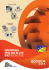• SV/PLUS: Multi-blade impeller for all models • SV/ECO: Backward-curved impeller for all models • Standard flanged inlet and outlet to aid installation on duct • They are supplied with 4 base ...

### IN-LINE DUCT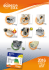• HEPT: Sheet steel cased casing long-cased fans (HEPT), with • H

### low-pressure centrifugal fans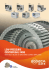CBD-1919-4M 1/5 CBD-1919-6M 1/10 CBD-2525-4M 1/2 CBD-2525-4M 3/4 CBD-2525-6M 1/5 CBD-2525-6M 1/3 CBD-2828-4M 1/2 CBD-2828-4M 3/4 CBD-2828-6M 1/3 CBD-2828-6M 3/4 CBD-3333-6T 1 1/2 CBD-333...